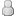• 11 Votes - 4.64 Average
• 1
• 2
• 3
• 4
• 5
11-12-2021, 03:52 PMPost: #372
 LunaMooPosting FreakPosts: 1,770 Joined: Sep 2013 Reputation: 159
```const float customX = 4.0; //edit only values in here const float customY = 3.0; // here const float zoom = 3.0; // and here, through you can set automatic zoom based on X or Y few lines lower const float amount = 0.60; // suitable range = 0.00 - 1.00 const float power = 0.5; // suitable range = 0.0 - 1.0 void main() {     float zoomByX = 16.0 / customX;     float zoomByY = 9.0 / customY;     float zoomByXY = zoom;          //zoomByXY = zoomByX;     // uncomment the above or below(remove // before the code) to have it zoom based on X or Y edge instead of the zoom you manually typed in above     //zoomByXY = zoomByY;     float xdivision = 1.0 / (customX / 16.0 * zoomByXY);     float ydivision = 1.0 / (customY / 9.0 * zoomByXY);     float2 vCoord0 = GetCoordinates().xy;     float3 color = Sample().xyz;     color = SampleLocation(float2(vCoord0.x * xdivision - (0.5 + xdivision / 2.0 - 1.0), vCoord0.y * ydivision - (0.5 + ydivision / 2.0 - 1.0)).xy).xyz;     float4 sum = vec4(0);     float3 bloom;     float2 adjustedCoords = float2(vCoord0.x * xdivision - (0.5 + xdivision / 2.0 - 1.0), vCoord0.y * ydivision - (0.5 + ydivision / 2.0 - 1.0)).xy;     for(int i= -3 ;i < 3; i++)     {         sum += SampleLocation(adjustedCoords + vec2(-1, i) * 0.004) * amount;         sum += SampleLocation(adjustedCoords + vec2( 0, i) * 0.004) * amount;         sum += SampleLocation(adjustedCoords + vec2( 1, i) * 0.004) * amount;     }     if (color.r < 0.3 && color.g < 0.3 && color.b < 0.3) {         bloom = sum.xyz * sum.xyz * 0.012 + color;     } else {         if (color.r < 0.5 && color.g < 0.5 && color.b < 0.5) {             bloom = sum.xyz * sum.xyz * 0.009 + color;         } else {             bloom = sum.xyz * sum.xyz * 0.0075 + color;         }     }     // Remove color bleed outside of the game scene caused by shrinking     float rangex = (0.5 + (xdivision / 2.0 - 1.0)) / xdivision;     float rangey = (0.5 + (ydivision / 2.0 - 1.0)) / ydivision;     if (vCoord0.x < rangex || vCoord0.x > 1.0 - rangex || vCoord0.y < rangey || vCoord0.y > 1.0 - rangey) {         bloom.xyz = float3(0.0,0.0,0.0).xyz;     }     SetOutput(float4(bloom.rgb, 1.0)); }```